# Demystifying delayed aesthetic evaluation: Part 1

Exploring the logic of `after_stat()` to peek inside ggplot internals

June Choe (University of Pennsylvania Linguistics)https://live-sas-www-ling.pantheon.sas.upenn.edu/
03-17-2022

This is a developing series of blog posts, scheduled for three parts:

## Introduction

The version 3.3.0 of {ggplot2} released back in early 2020 introduced a family of functions for finer control over mapping variables to aesthetics: `after_stat()`, `after_scale()`, and `stage()`.

The big idea behind these functions is that layers sometimes derive new variables from the raw data that they receive, and so ggplot should offer a way of letting the user access with these internally-computed variables inside the `aes()`.

While this may sound esoteric, the mapping of an internal variable to an aesthetic is ubiquitous, both in `{ggplot2}` itself as well as in many extension packages. For example, we take it for granted that layers like `geom_bar()` and `geom_histogram()` draw bars in the x/y-coordinate space and yet require just a single mapping to one of the two aesthetics.

``````library(ggplot2)
theme_set(theme_classic(14))

penguins <- na.omit(palmerpenguins::penguins)
rmarkdown::paged_table(penguins)
``````
``````ggplot(penguins, aes(x = bill_depth_mm)) +
geom_histogram()
ggplot(penguins, aes(x = species)) +
geom_bar()
``````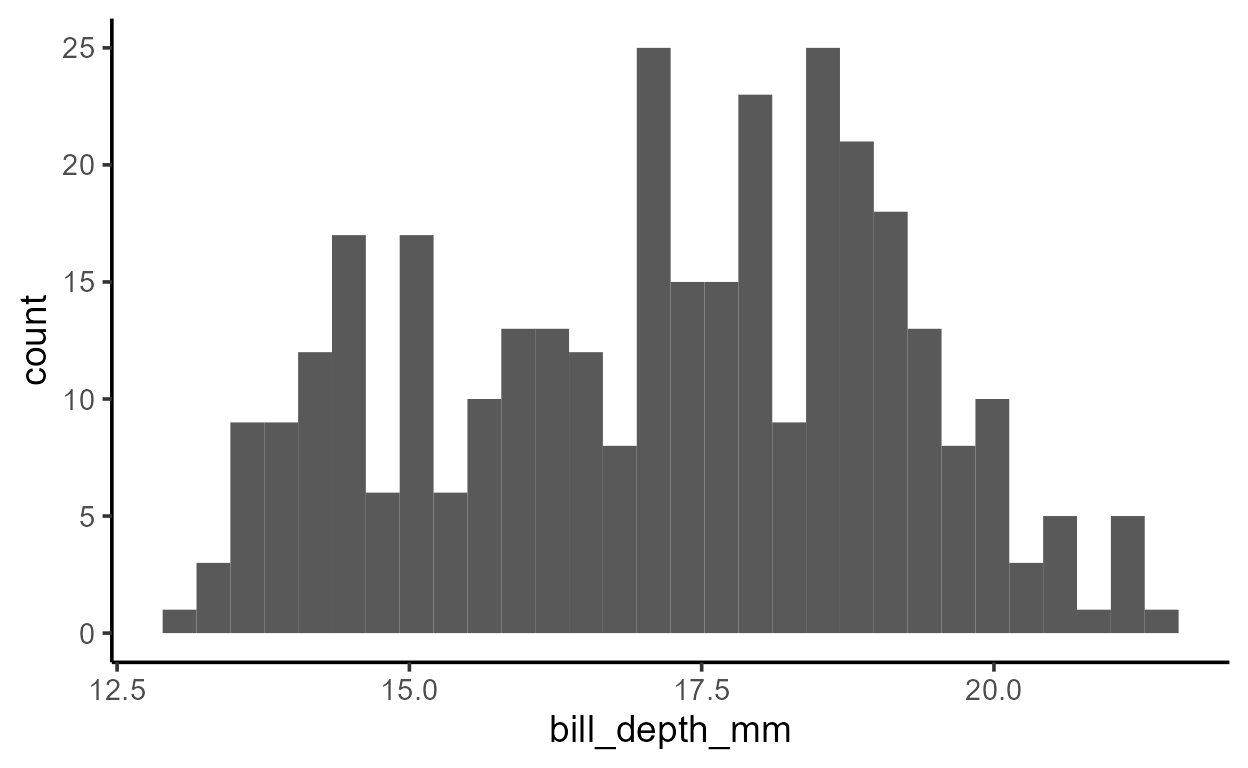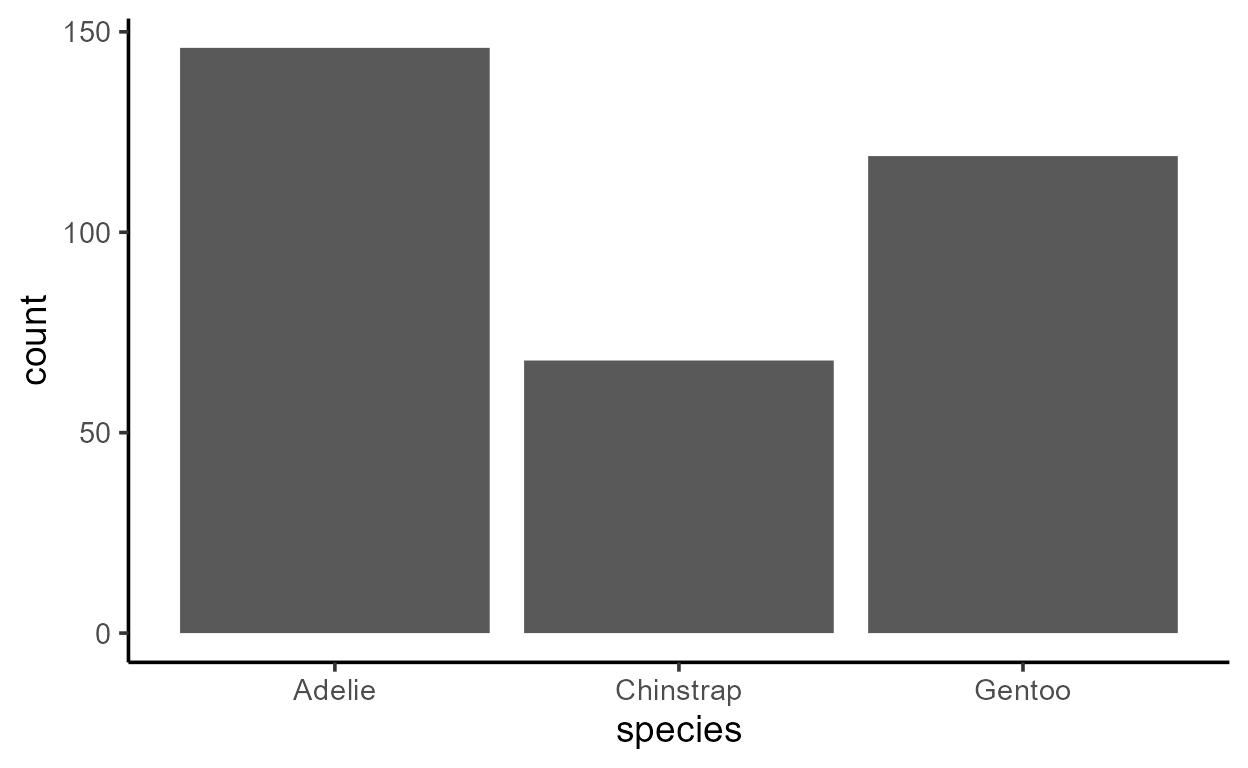Despite only specifying a mapping to `x`, there is clearly also a variable mapped to `y` in both plots above - a variable called count.

We can infer that the count variable is computed by the layer since it doesn’t exist in our raw data `penguins`:

``````"count" %in% colnames(penguins)
``````
``   FALSE``

Which also means that if we try to use `count` in `aes()` like we would any other column in our data, we get an error:

``````ggplot(penguins, aes(species, y = count)) +
geom_bar()
``````
``  Error in FUN(X[[i]], ...): object 'count' not found``

But we don’t just have to infer that `count` is computed by the layer. We can see it from the output of a layer’s data transformation pipeline, which we can get with `layer_data()`.1 Here, we pass `layer_data()` our bar plot, which by default returns us the data for the first layer:

``````p <- ggplot(penguins, aes(x = species)) +
geom_bar()
layer_data(p, i = 1L) # argument `i` specifies the layer index
``````
``````      y count prop x flipped_aes PANEL group ymin ymax xmin xmax colour   fill
1 146   146    1 1       FALSE     1     1    0  146 0.55 1.45     NA grey35
2  68    68    1 2       FALSE     1     2    0   68 1.55 2.45     NA grey35
3 119   119    1 3       FALSE     1     3    0  119 2.55 3.45     NA grey35
size linetype alpha
1  0.5        1    NA
2  0.5        1    NA
3  0.5        1    NA``````

There’s a lot going on in this dataframe but pay attention to two things:

• There is a new column called `count`
• The `y` column is the same as `count`

Both of these must happen for our plots above to have `count` mapped to `y`.

But how does ggplot know to wait until `count` is computed to map it to `y`?

## `after_stat()`

The technical details of how and where `count` is computed will be covered in the Part 2 of this series. For now, all we need to know is that layers like `geom_bar()` and `geom_histogram()` transforms the raw data that it receives to derive new values like `count`.

So here’s the big idea: to allow aesthetics to be mapped to internally calculated variables like `count`, there must be a way to delay some aesthetic mappings.

More specifically, when it comes to variables like `count`, we need a way to reference it after the statistical transformation has taken place internally. As you might have guessed, we can do this with the transparently-named function `after_stat()`! It takes one argument `x` which is captured as an expression, to be evaluated later.

So in our case, the fact that `count` is mapped to `y` after the statistical transformation takes place suggests that `y` is mapped to `after_stat(count)`. This is indeed the case, and we can make this default mapping explicit in the `aes()`:

``````ggplot(penguins, aes(species, y = after_stat(count))) +
geom_bar()
``````The expression inside `after_stat()` is evaluated in what’s called a data-masked context, much like in `aes()` or `{dplyr}` verbs like `mutate()` and `summarize()`. This is why you can just refer to `count` as if it were a variable name and `after_stat()` will grab that column vector from the transformed data.

But now we’re faced with another question: what is the transformed data that `after_stat()` looks for variables in? We know it’s a version of our original data, but what does the output actually look like?

To help us answer this question I’m going to introduce a function called `inspect_after_stat()`. I’ll leave the code here for reproducibility purposes but it’s supposed to be a bit mysterious right now, so don’t read too much into it!

`inspect_after_stat`

No dependency version:

``````#' Inspect a layer's data after computation by the Stat
#'
#' @param p A `ggplot` object
#' @param i An integer. The position of the layer's data to return.
#'
#' @return A dataframe
inspect_after_stat <- function(p, i = 1L) {
._env <- environment()
.out <- NULL
suppressMessages({
trace(
what = "ggplot_build.ggplot",
tracer = substitute(assign(".out", data[[i]], envir = ._env), ._env),
at = 19L,
print = FALSE,
where = asNamespace("ggplot2")
)
})
ggplot_build(p)
suppressMessages({
untrace("ggplot_build.ggplot", where = asNamespace("ggplot2"))
})
.out
}
``````
##### Technical details:

`inspect_after_stat()` wraps around `base::trace()`, a debugging function which can inject expressions to be evaluated at a specific position of a function when it is called. The function being traced is the S3 method `ggplot_build()` as defined for class `<ggplot>`. The function evaluates and logs the value of `data[[i]]` at Step 19 of `ggplot_build.ggplot`. This is the value of the `i`th layer’s data right after the statistical transformation happens in the preceding step, Step 18:

``````# https://github.com/tidyverse/ggplot2/blob/main/R/plot-build.r#L72
body(ggplot2:::ggplot_build.ggplot)[]
``````
``  data <- by_layer(function(l, d) l\$compute_statistic(d, layout))``

Part 2 will explore what happens here in more detail.

If you’re interested in more functions like `inspect_after_stat()` that allow you to interact with ggplot internals, take a look at my package `{ggtrace}`!

``````# {ggtrace} version, not run
inspect_after_stat <- function(p, i = 1L) {
out <- ggtrace::ggtrace_inspect_vars(
x = p,
method = ggplot2:::ggplot_build.ggplot,
at = 19L,
vars = "data"
)
out[][[i]]
}
``````

We’ll use `inspect_after_stat()` to “peak inside” ggplot internals and inspect the state of a layer’s data after the statistical computation has taken place.

Let’s start with a simple example of `geom_bar()`:

``````p_bar <- ggplot(penguins, aes(species)) +
geom_bar(aes(fill = species))
p_bar
``````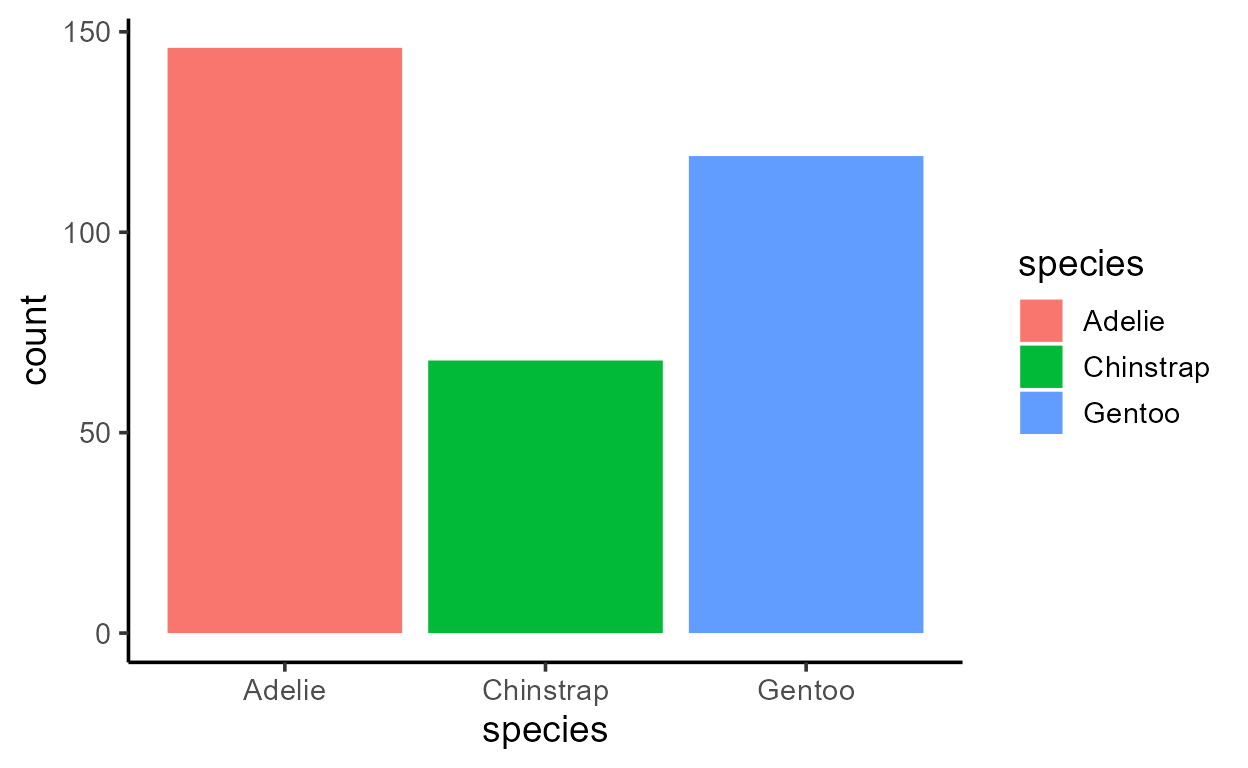When we inspect the state of the data for the first (and only) layer of our plot after the statistical transformation, it looks like the following:

``````inspect_after_stat(p_bar)
``````
``````    count prop x width flipped_aes      fill PANEL group
1   146    1 1   0.9       FALSE    Adelie     1     1
2    68    1 2   0.9       FALSE Chinstrap     1     2
3   119    1 3   0.9       FALSE    Gentoo     1     3``````

At this stage, the data is collapsed such that each row contains information about one bar.

Notice how the statistical transformation done by `geom_bar()` is basically just `dplyr::count()`:

``````library(dplyr)
penguins %>%
count(species)
``````
``````  # A tibble: 3 x 2
species       n
<fct>     <int>
2 Chinstrap    68
3 Gentoo      119``````

It’s `{dplyr}` all the way down!2

If we take the notion of delayed aesthetic evaluation seriously, then all columns in the dataframe returned by `inspect_after_stat()` should be accessible with `after_stat()`. Indeed this is the case.

To demonstrate, I can map `y` to each variable in the transformed data, converting to numeric as needed to conform to the plot’s continuous y scale.

``````# Plots are printed left -> right and top -> bottom
# in order of `matrix(1:8, ncol = 2, byrow = TRUE)`
p_bar + aes(y = after_stat(count))
p_bar + aes(y = after_stat(prop))
p_bar + aes(y = after_stat(x))
p_bar + aes(y = after_stat(width))
p_bar + aes(y = after_stat(as.numeric(flipped_aes)))
p_bar + aes(y = after_stat(nchar(as.character(fill))))
p_bar + aes(y = after_stat(as.numeric(PANEL)))
p_bar + aes(y = after_stat(group))
``````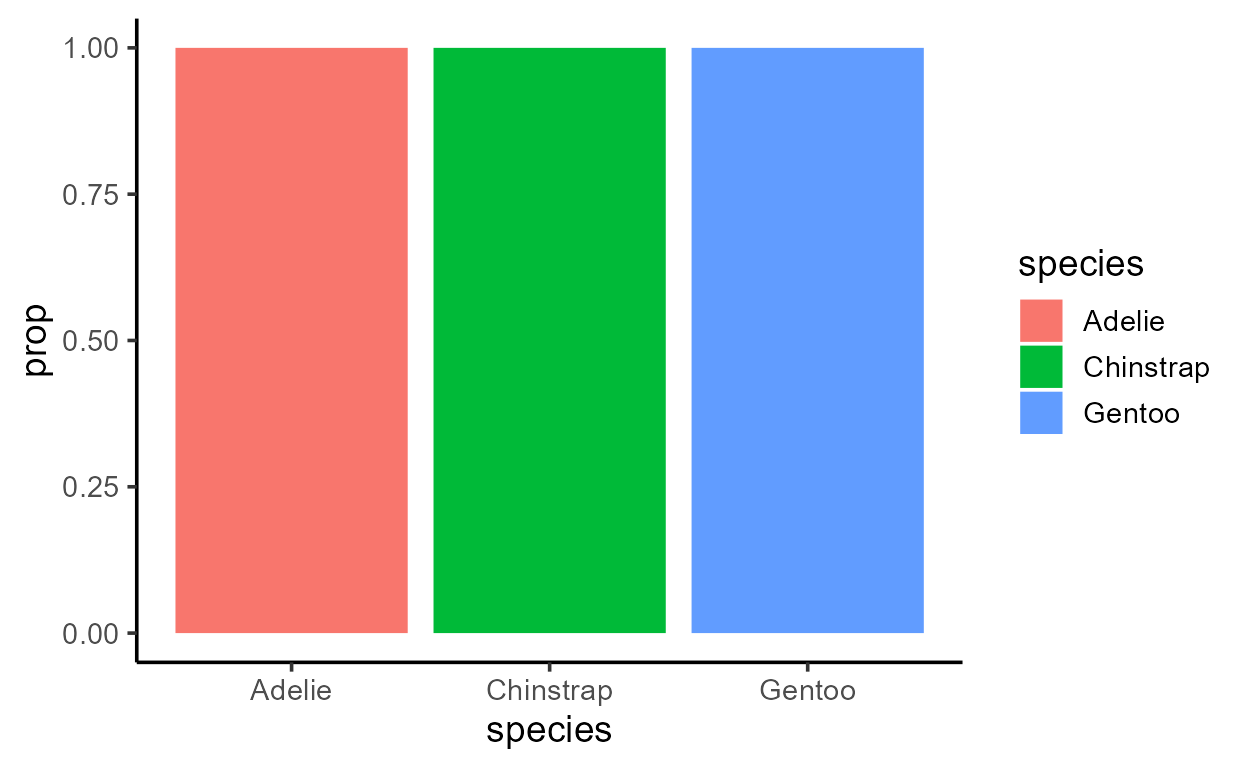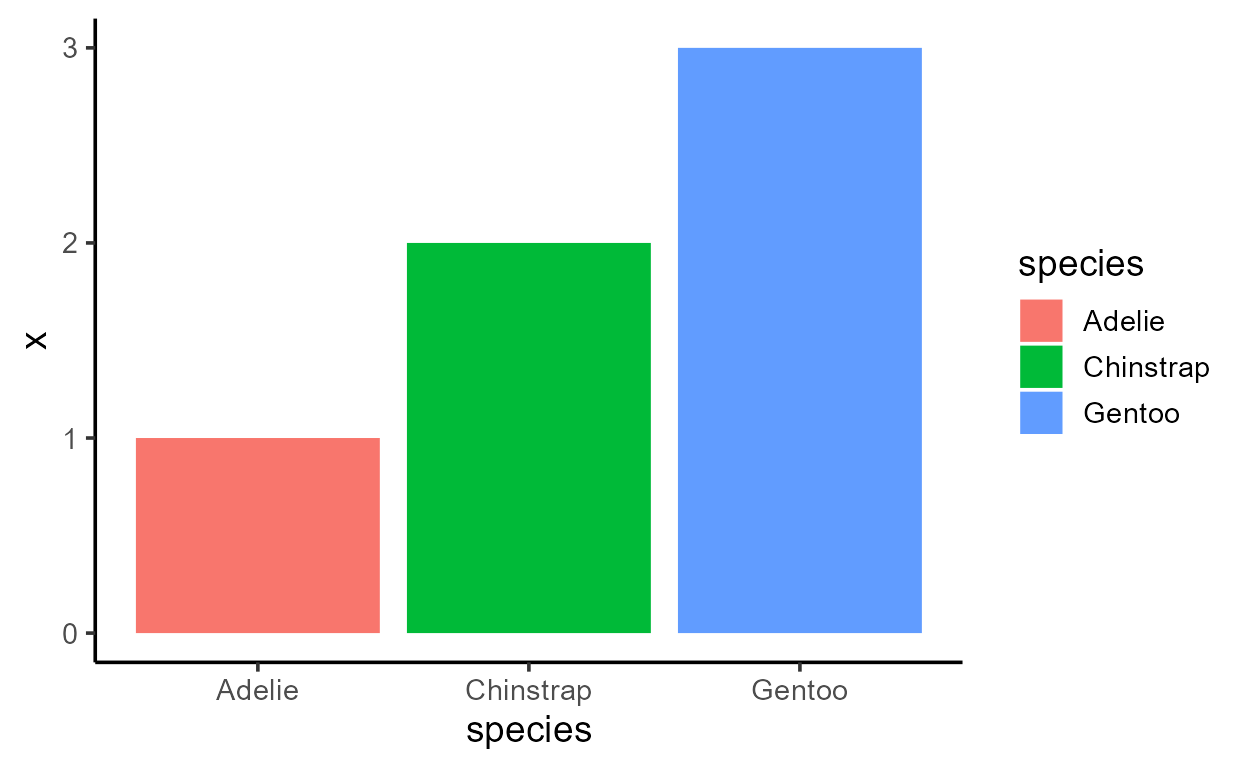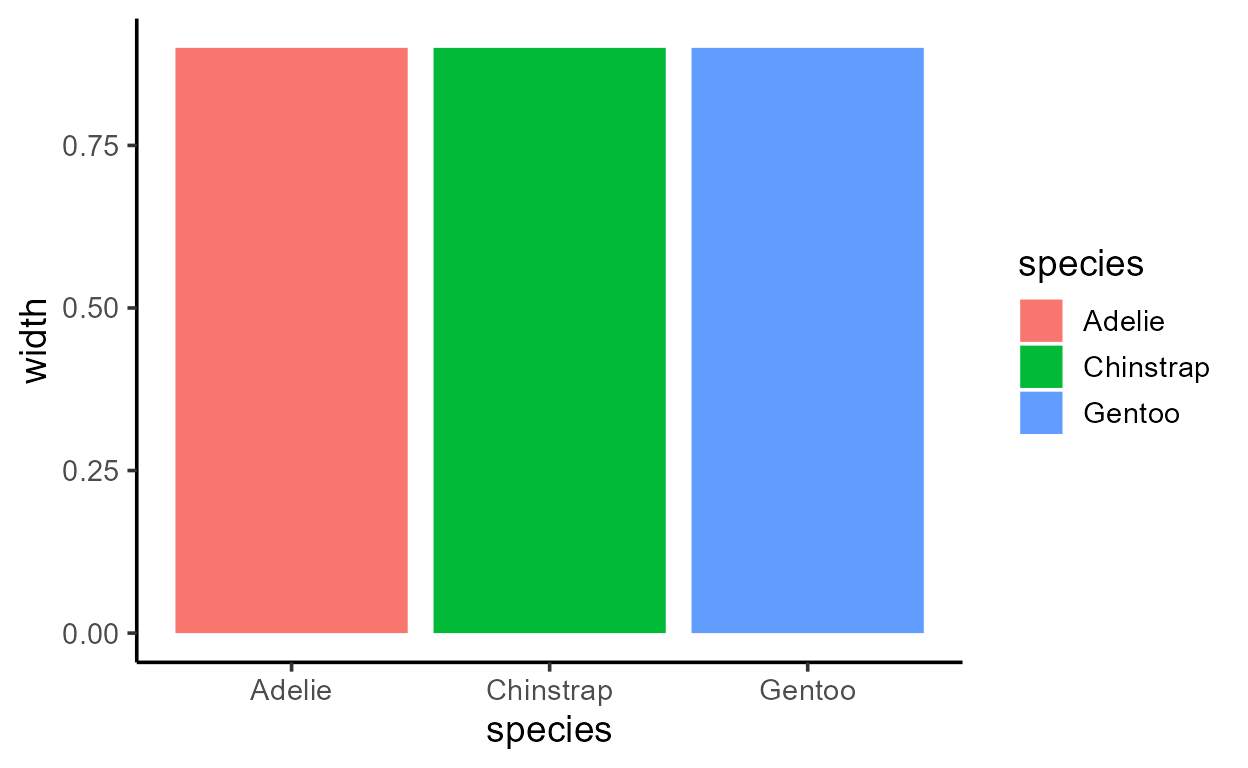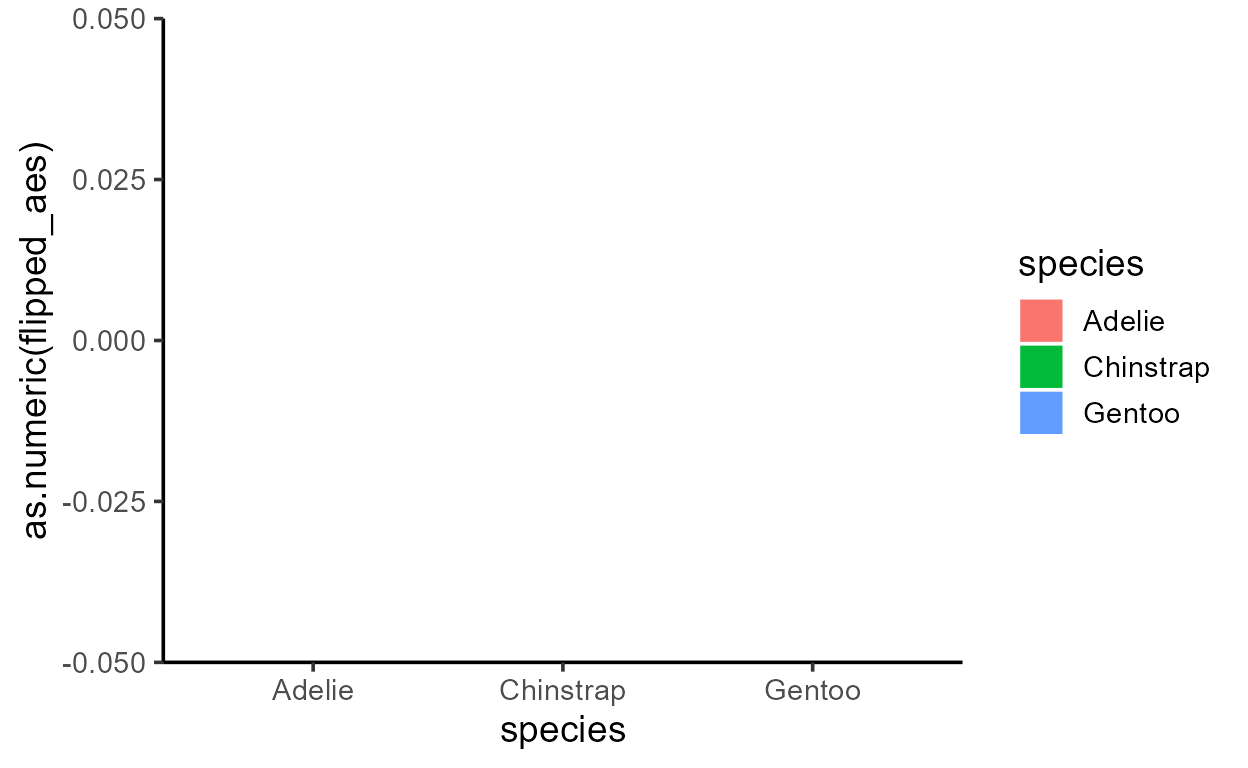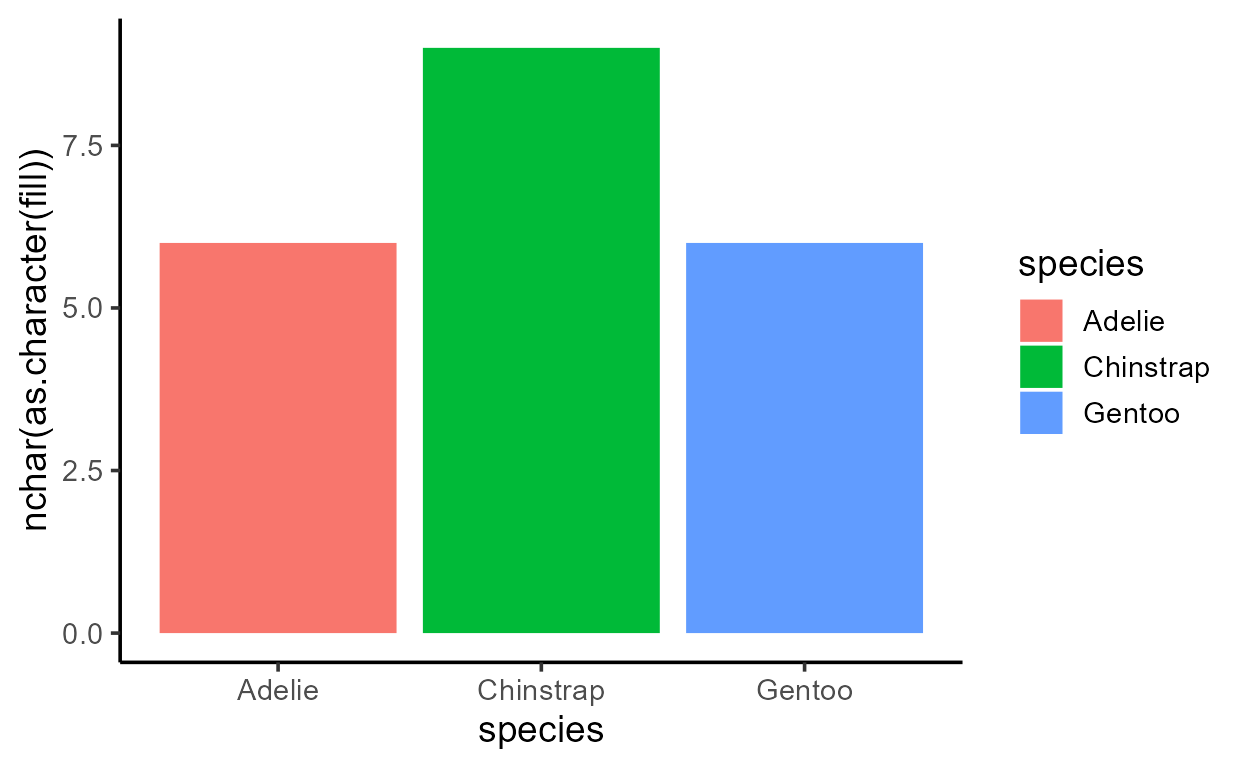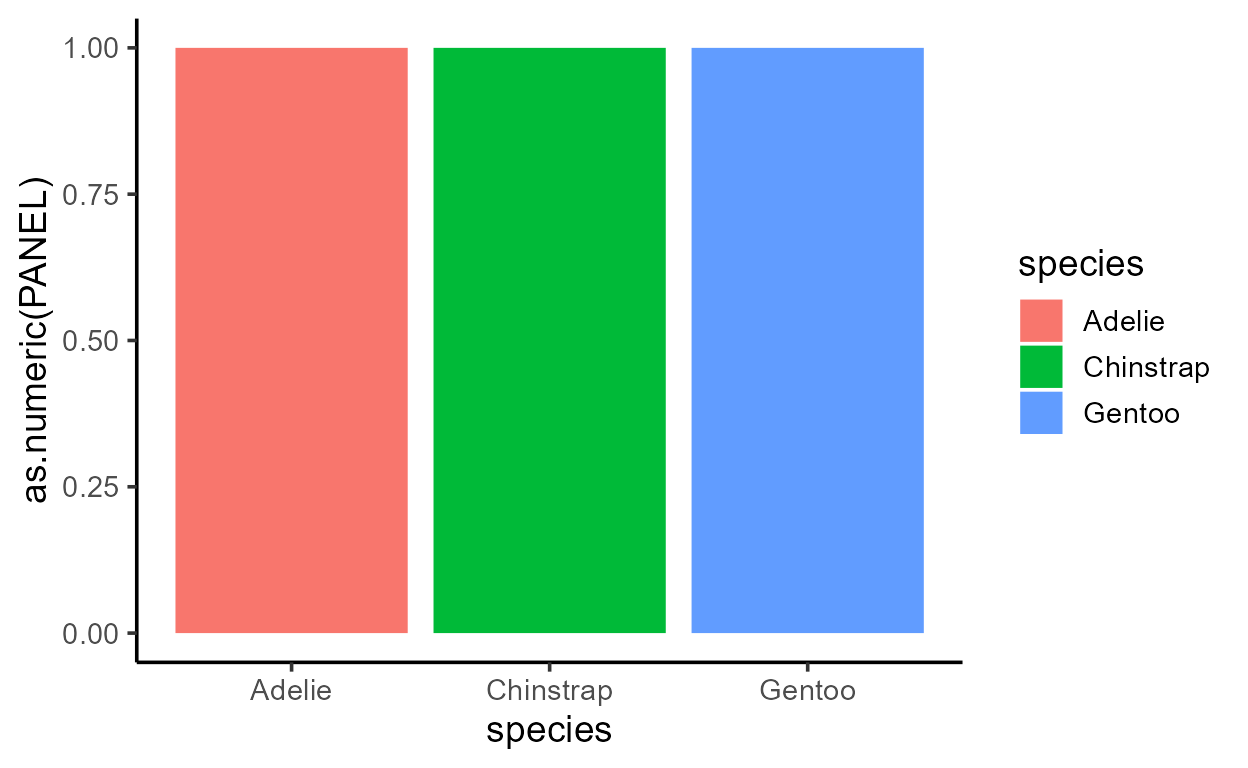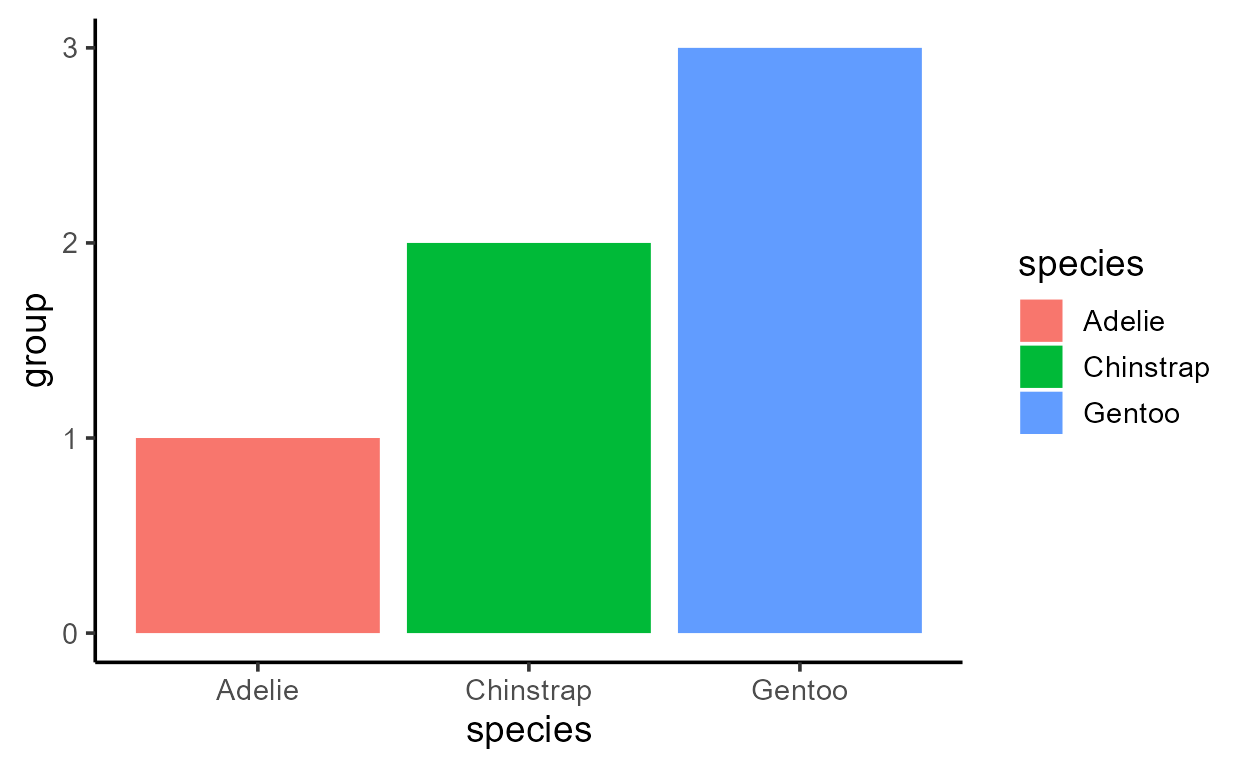Before I get sacked, I should add that just because you can, doesn’t mean you should! More specifically, `after_stat()` should only really be used to access variables computed from the stat stage like `count` and `prop` (we’ll go over how to tell what variables are from the stat stage in Part 2). The stat transformation is just one of many transformations that the data goes through to become drawing-ready, and so the state of the “after stat” data also carries the output of other processes that came before it like facet-ing and positional scale transformations which we shouldn’t touch.

And in fact, many more transformations happen after the stat stage as well, so it’s neither the first nor the last thing to happen to the data. Using `layer_data()` again, we see that the final form of the data builds on our data from that we saw from the stat stage:

``````layer_data(p_bar)
``````
``````       fill   y count prop x flipped_aes PANEL group ymin ymax xmin xmax colour
1 #F8766D 146   146    1 1       FALSE     1     1    0  146 0.55 1.45     NA
2 #00BA38  68    68    1 2       FALSE     1     2    0   68 1.55 2.45     NA
3 #619CFF 119   119    1 3       FALSE     1     3    0  119 2.55 3.45     NA
size linetype alpha
1  0.5        1    NA
2  0.5        1    NA
3  0.5        1    NA``````

Lastly, note that while additional variables in `layer_data()` like `size` is technically also from after the stat stage, they aren’t accessible in the `after_stat()`:

``````p_bar + aes(y = after_stat(size))
``````
``  Error in after_stat(size): object 'size' not found``

This is because `after_stat()` references a snapshot of the data right after the stat transformation, which is the data we saw with `inspect_after_stat()`, repeated below. Notice how the `size` column is not yet present at this stage:

``````inspect_after_stat(p_bar)
``````
``````    count prop x width flipped_aes      fill PANEL group
1   146    1 1   0.9       FALSE    Adelie     1     1
2    68    1 2   0.9       FALSE Chinstrap     1     2
3   119    1 3   0.9       FALSE    Gentoo     1     3``````

## Another practice with `prop`

Let’s look at another example of `after_stat()` to drive the point home.

You might have noticed in the after stat data how the internally computed `prop` column just has the value 1, which isn’t too informative when we plot it:

``````ggplot(penguins, aes(species)) +
geom_bar(aes(y = after_stat(prop)))
``````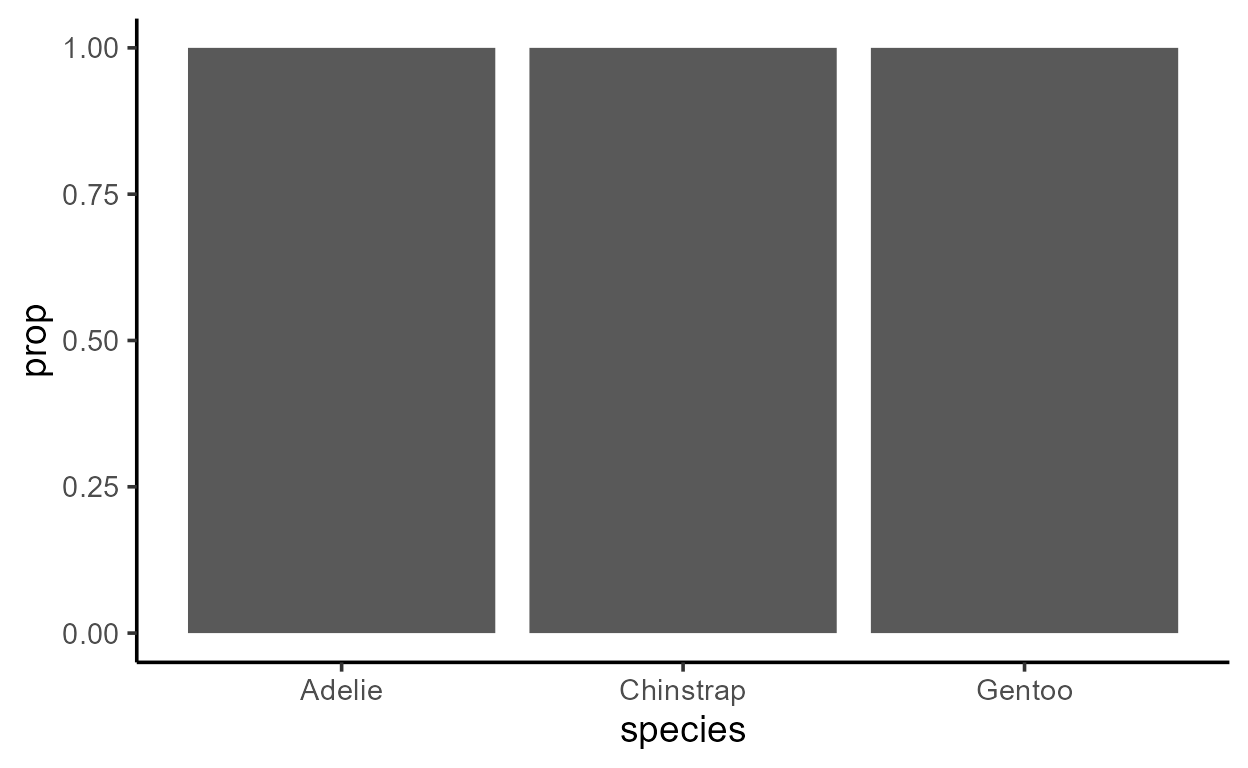What is `prop` and when does it ever take on a different value?

If you look at the documentation for `geom_bar()` under the section “Computed variables”, you will see the following descriptions for the two computed variables `count` and `prop`:

• count: number of points in the bin
• prop: groupwise proportion

The value of `prop` was 1 for `p_bar` because each bar was assigned a unique group as indicated by the values of the `group` column, so of course the computation of groupwise proportion was uninteresting!

If we want to change that, we’d need to explicitly specify a `group`-ing that creates fewer groups than the number of bars present.

In this example, we have `geom_bar()` make a bar for each category of `species` as mapped to `x`, but internally represent the three bars as belonging to one of two groups - the “Adelie group” and the “not-Adelie group”:

``````p_bar_prop <- ggplot(penguins, aes(species)) +
geom_bar(
aes(
y = after_stat(prop),
group = species == "Adelie" # Assign the 3 bars into 2 groups
)
)
p_bar_prop
``````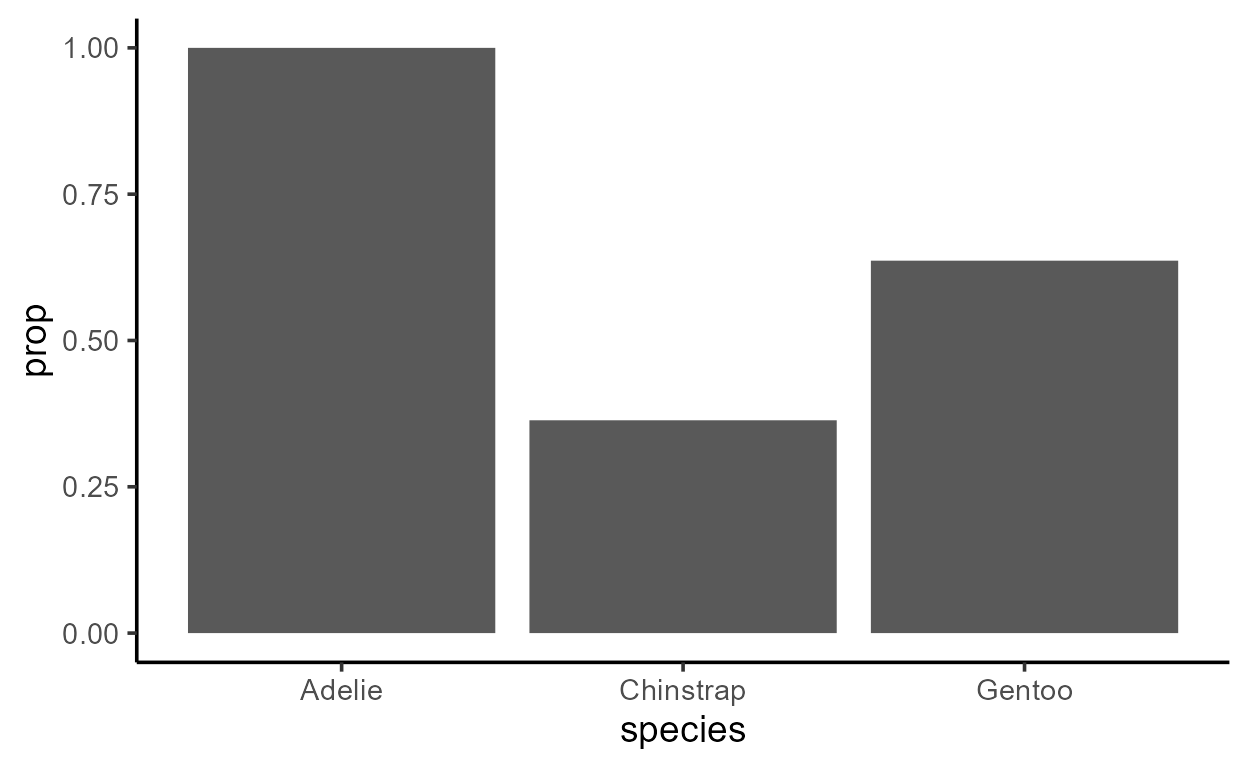It doesn’t look like there are two groups underlyingly, but let’s see what happens when we inspect the data transformed by the Stat:

``````inspect_after_stat(p_bar_prop)
``````
``````    count      prop x width flipped_aes group PANEL
1    68 0.3636364 2   0.9       FALSE     1     1
2   119 0.6363636 3   0.9       FALSE     1     1
3   146 1.0000000 1   0.9       FALSE     2     1``````

We see only two groups (values of `group` are either 1 or 2), as we expect. And where two bars (rows) belong to the same group, the values of `prop` add up to 1!

Our `p_bar_prop` has grey fill for all bars that make the grouping structure visually ambiguous, but that was for expository purposes only. If we wanted to fill the bars by group, we could do it in at least two ways.

A hacky way that is 100% not recommended but possible is to mark the groupings post-hoc, by grabbing the `group` variable in the `after_stat()` and mapping that to something like `fill`:

``````p_bar_prop +
aes(fill = after_stat(as.factor(group)))
``````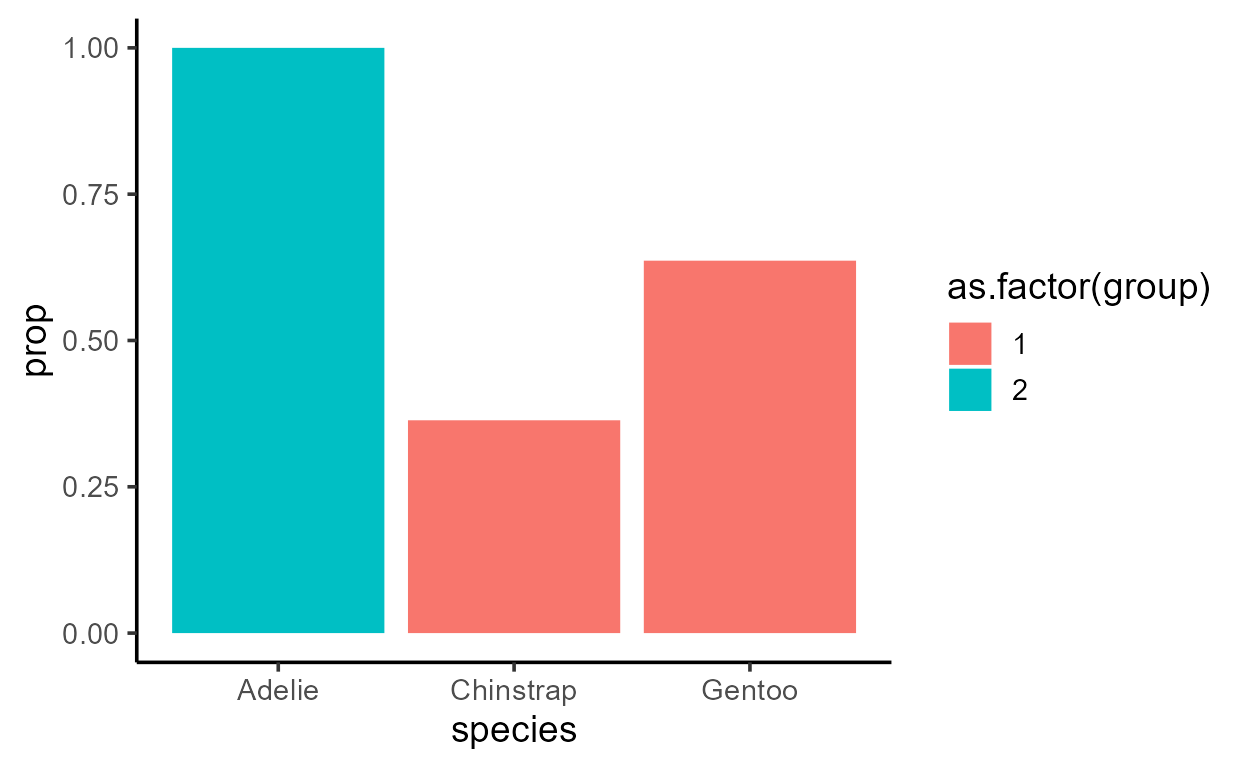This is dangerous not only because it adds an unnecessary line after `geom_bar()`, but also because it uses `+ aes()` to map an `after_stat()` variable globally. You should avoid this because different layers do different statistical transformations (we’ll discuss this shortly in the next section), and that can lead to surprising behaviors like this:3

``````p_bar_prop +
aes(fill = after_stat(as.factor(group))) +
# This next line does something unexpected to the fill!
geom_label(aes(x = 2, y = 0.5, label = "Hello"), stat = "unique")
``````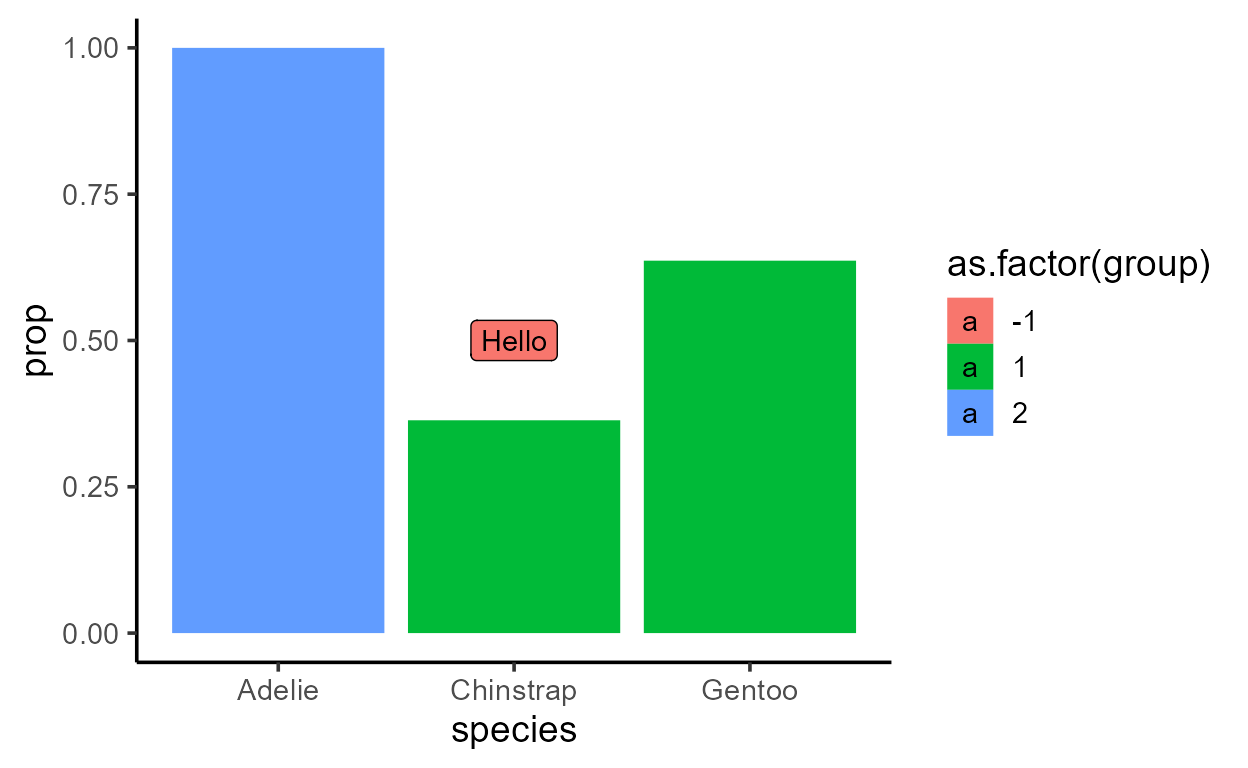A safer, more principled way is to go back inside the `aes()` for the bar layer and visually mark the underlying grouping there:

``````p_bar_prop_group_fill <- ggplot(penguins, aes(species)) +
geom_bar(
aes(
y = after_stat(prop),
fill = species == "Adelie"   #< Here!
)
)
p_bar_prop_group_fill
``````For a quick experiment, note what happens if you only map `species == "Adelie"` to `fill`:

``````p_bar_prop_only_fill <- ggplot(penguins, aes(species)) +
geom_bar(
aes(
y = after_stat(prop),
# group = species == "Adelie",
)
)
p_bar_prop_only_fill
``````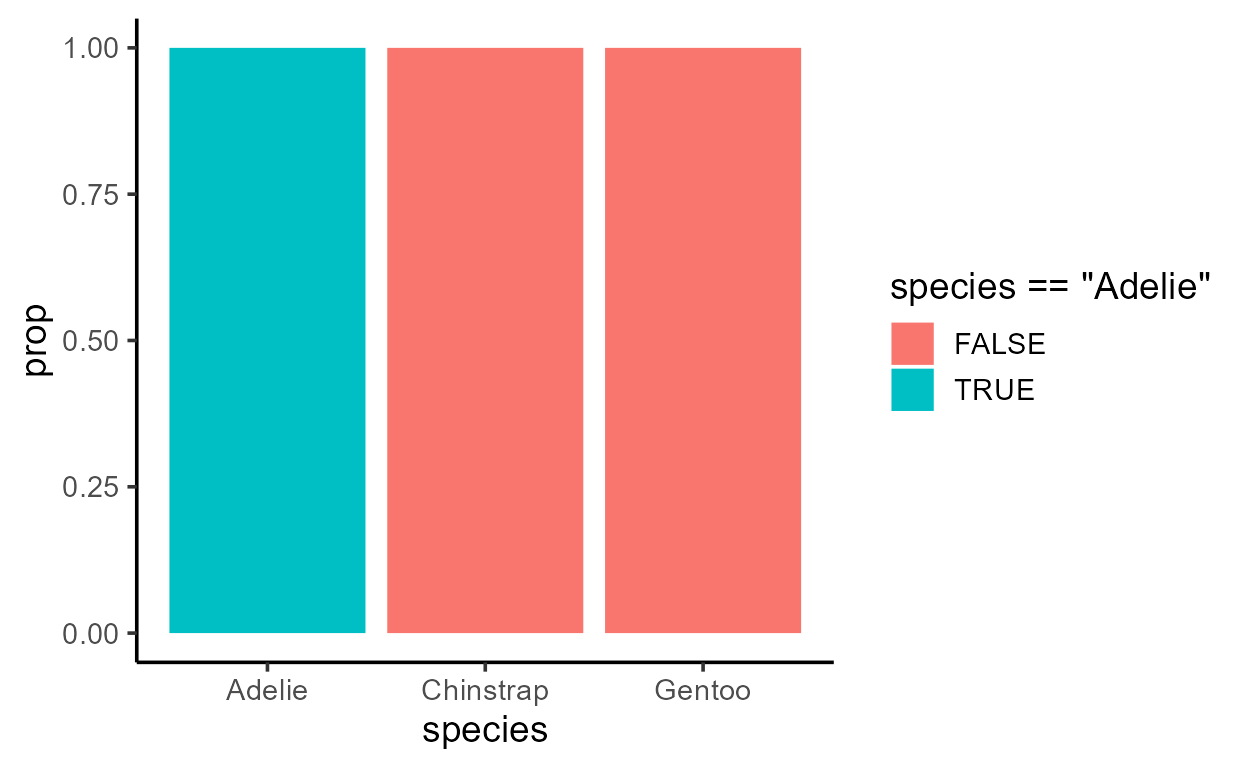As you might have guessed, we get a different plot because the underlying grouping structure is different. See how this does not change the value of `count` (absolute) but does change the value of `prop` (relative):4

``````# 2 groups present
inspect_after_stat(p_bar_prop_group_fill)
``````
``````    count      prop x width flipped_aes  fill group PANEL
1    68 0.3636364 2   0.9       FALSE FALSE     1     1
2   119 0.6363636 3   0.9       FALSE FALSE     1     1
3   146 1.0000000 1   0.9       FALSE  TRUE     2     1``````
``````# 3 groups present
inspect_after_stat(p_bar_prop_only_fill)
``````
``````    count prop x width flipped_aes  fill PANEL group
1    68    1 2   0.9       FALSE FALSE     1     1
2   119    1 3   0.9       FALSE FALSE     1     2
3   146    1 1   0.9       FALSE  TRUE     1     3``````

Let’s conclude this section by putting the issue of practicality back on the table.

The most common usecase for `prop` is to use it in conjunction with something like `group = 1`5 to “normalize” the y-scale within each facet. This is useful when the data has unbalanced samples across panels but you want to emphasize the relative distribution of categories in the x-axis within each panel:

``````# Absolute value `count` mapped to `y`
ggplot(penguins, aes(species)) +
geom_bar(aes(y = after_stat(count))) +
facet_grid(~ island, scales = "free_x", space = "free")
``````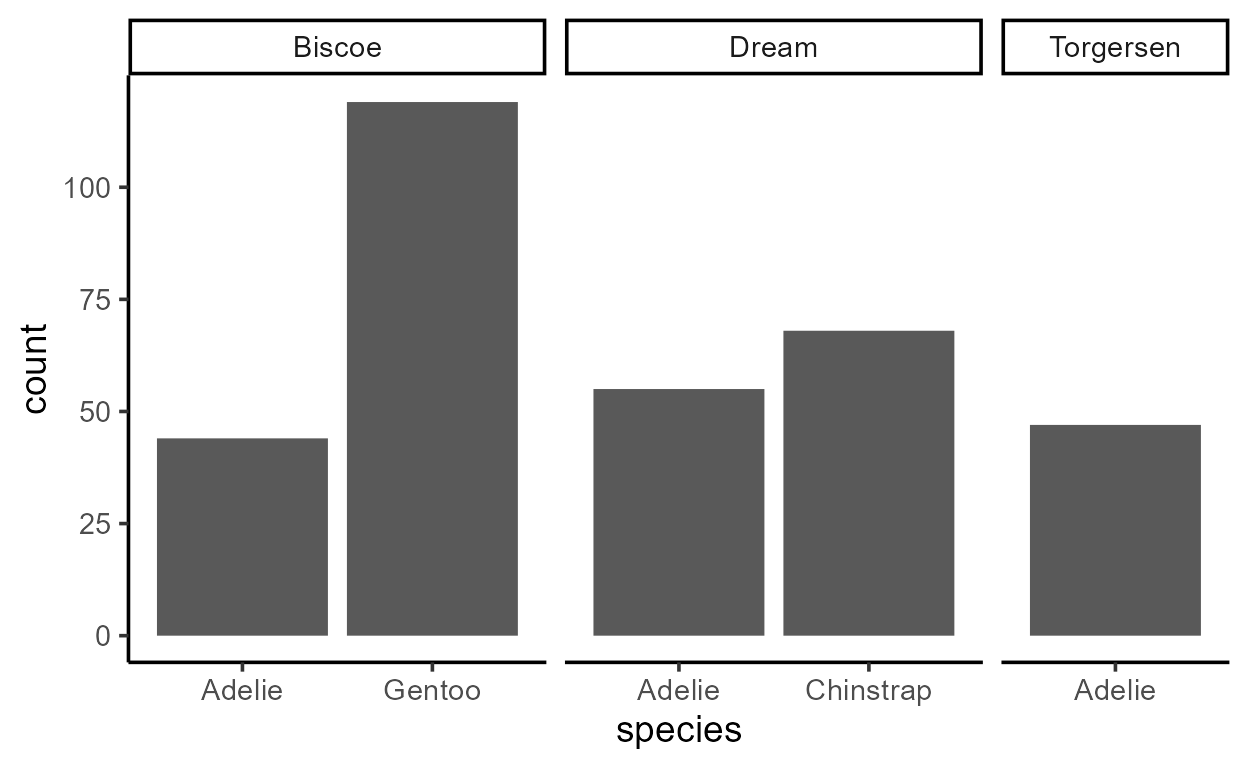``````# Relative value `prop` mapped to `y`
ggplot(penguins, aes(species)) +
geom_bar(aes(y = after_stat(prop), group = 1)) +
facet_grid(~ island, scales = "free_x", space = "free")
``````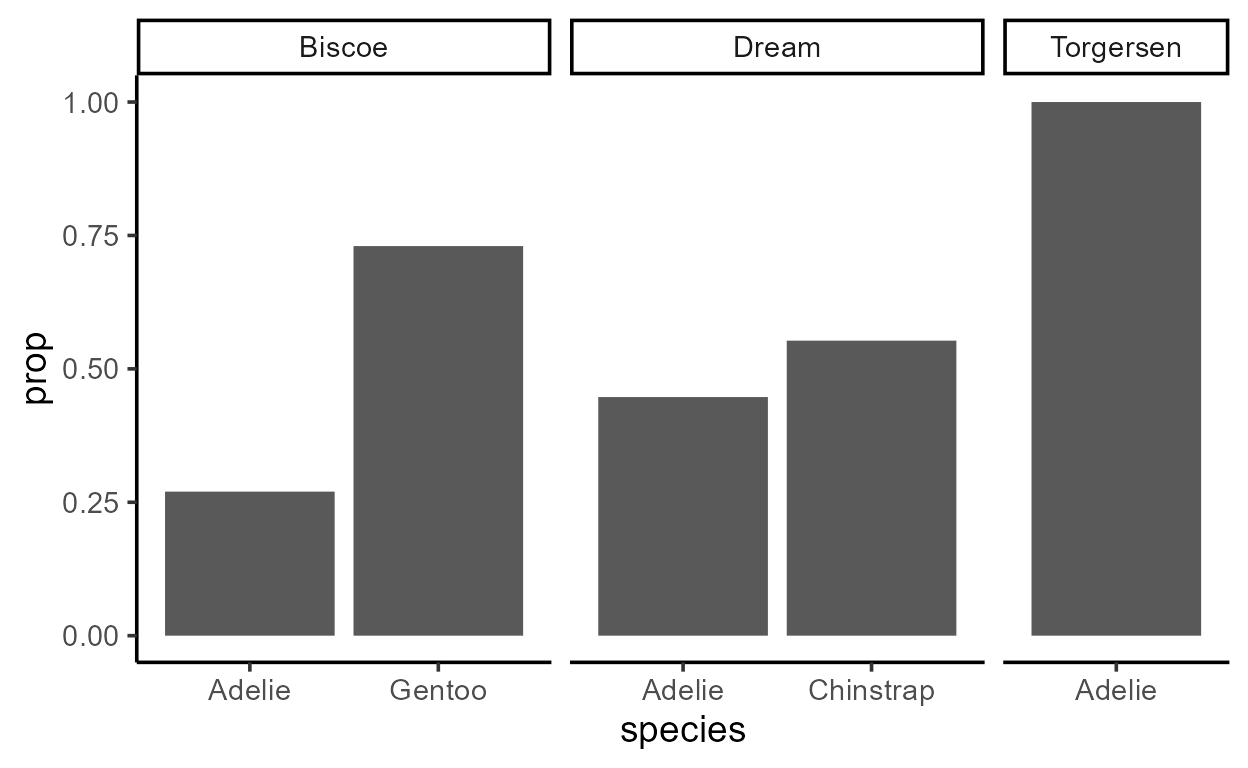We see that `aes(group = 1)` had the effect of making sure that `prop` adds up to 1 within each facet because all bars in a panel share the same group.6

## Baby steps to the Stat ggproto

### Same geom, different stats

One thing that you might have noticed throughout this exercise is that it’s not transparent from the name `geom_bar()` that this layer is going to compute internal variables like `count` and `prop` and use a default y-aesthetic of `after_stat(count)`.

This is responsible for one of the most enduring points of confusion in ggplot: the difference between `geom_col()` and `geom_bar()`.

Whether you call the rectangle a “column” or a “bar” sounds like silly semantics, so why do they behave so differently?

``````ggplot(penguins, aes(species)) +
geom_col() # but works with `geom_bar()`
``````
``````  Error in `check_required_aesthetics()`:
! geom_col requires the following missing aesthetics: y``````
``````penguins %>%
count(species) %>%
ggplot(aes(species, n)) +
geom_bar() # but works with `geom_col()`
``````
``````  Error in `f()`:
! stat_count() can only have an x or y aesthetic.``````

You get a small hint in the error message for the `geom_bar()` example - it complains that `stat_count()` can only have an x or y aesthetic.

But we’ve never said anything about `stat_count()` - all we have is a layer for `geom_bar()`!

Well it turns out that `geom_bar()` and `stat_count()` are two sides of the same coin. Both these functions return a layer that has a “bar” geom a “count” stat.7

``````geom_bar()
``````
``````  geom_bar: width = NULL, na.rm = FALSE, orientation = NA
stat_count: width = NULL, na.rm = FALSE, orientation = NA
position_stack``````
``````stat_count()
``````
``````  geom_bar: na.rm = FALSE, orientation = NA, width = NULL
stat_count: na.rm = FALSE, orientation = NA, width = NULL
position_stack``````

With that in mind, let’s return to the code for our penguin species bar plot:

``````ggplot(penguins, aes(species)) +
geom_bar()
``````We’ve said that `geom_bar()`, by default, has a mapping of `aes(y = after_stat(count))`, so let’s make that explicit again:

``````ggplot(penguins, aes(species)) +
geom_bar(
aes(y = after_stat(count))
)
``````

And as we just saw, `geom_bar()` also uses a “count” stat by default, so let’s make that explicit as well:

``````ggplot(penguins, aes(species)) +
geom_bar(
aes(y = after_stat(count)),
stat = "count"
)
``````

Hopefully the pieces are starting to come together: the computation of the internal variable `count` isn’t about the “bar” geom - it’s about the “count” stat.

So `geom_col()` errors with `after_stat(count)` not because it’s drawing something different (it’s not!), it’s just calculating something different. In more technical terms, `geom_col()` doesn’t use `stat = "count"` like `geom_bar()` does. It uses `stat = "identity"` instead, which does an “identity” transformation on the data (i.e., leaves it alone):

``````geom_col()
``````
``````  geom_col: width = NULL, na.rm = FALSE
stat_identity: na.rm = FALSE
position_stack``````

Therefore, no new variables get calculated inside `geom_col()`. In fact, the data before and after the statistical transformation look nearly identical:

``````penguins_counted <- penguins %>%
count(species)

# The raw data
penguins_counted
``````
``````  # A tibble: 3 x 2
species       n
<fct>     <int>
2 Chinstrap    68
3 Gentoo      119``````
``````p_col <- penguins_counted %>%
ggplot(aes(species, n)) +
geom_col()

# The data after transformation
inspect_after_stat(p_col)
``````
``````    x   y PANEL group
1 1 146     1     1
2 2  68     1     2
3 3 119     1     3``````

What’s more, by overriding the `stat`, you can make `geom_bar()` behave like `geom_col()`:

``````p_bar_identity <- penguins_counted %>%
ggplot(aes(species, n)) +
geom_bar(stat = "identity") # `geom_bar()` no longer errors

# The data after transformation
inspect_after_stat(p_bar_identity)
``````
``````    x   y PANEL group
1 1 146     1     1
2 2  68     1     2
3 3 119     1     3``````

In sum, the variables that are available in the `after_stat()` is determined by what kind of `stat` a layer uses. And as we just saw, even if a layer is called a `geom_*()` it still has a `stat`. In fact, every layer has a stat and a geom, even though the functions that generate these layers have names like `stat_*()` and `geom_*()`.

It should be noted that the choice of a default `stat` for `geom_*()` layers can range from more transparent (like the pairing of `geom = "smooth"` and `stat = "smooth"` in `geom/stat_smooth()`) to less transparent (like `geom_col(stat = "identity")`). But it’s also worth keeping in mind that all defaults are carefully curated by the developers to improve user experience.8

### Same stat, different geoms

If all layers have a stat and a geom, and if `geom_*()` layers have default stats, then `stat_*()` layers must also have defaults geoms.

Indeed, this is the case. Remember the `stat_count()` layer from the error message earlier? Because it uses the “count” stat and has a default geom of “bar”, it works like `geom_bar()` right out of the box:

``````ggplot(penguins, aes(species)) +
stat_count()
``````And here are the defaults of `stat_count()` spelled out:

``````ggplot(penguins, aes(species)) +
stat_count(
aes(y = after_stat(count)),  # default mapping to `y`
geom = "bar"                 # default `geom`
)
``````

You might be wondering: why are there two ways of doing the same thing? Some people think its a design mistake but I appreciate the option because it can improve readability.

For example, we talked about how we can supply a different stat to a `geom_*()` layer like `geom_bar(stat = "identity")`. Actually, we can override the default geom/stat for any layer as long as we satisfy the required set of aesthetic mappings for that layer.

This allows us to do some nifty things like setting the stat of `geom_text()` to “count”, and then mapping the internally computed variable `count` to the `y` and `label` aesthetics to show the number of counts at the top of each bar:

``````ggplot(penguins, aes(species)) +
geom_bar() +
geom_text(
aes(y = after_stat(count), label = after_stat(count)),
stat = "count", vjust = -.4 # little vertical nudging with `vjust`
)
``````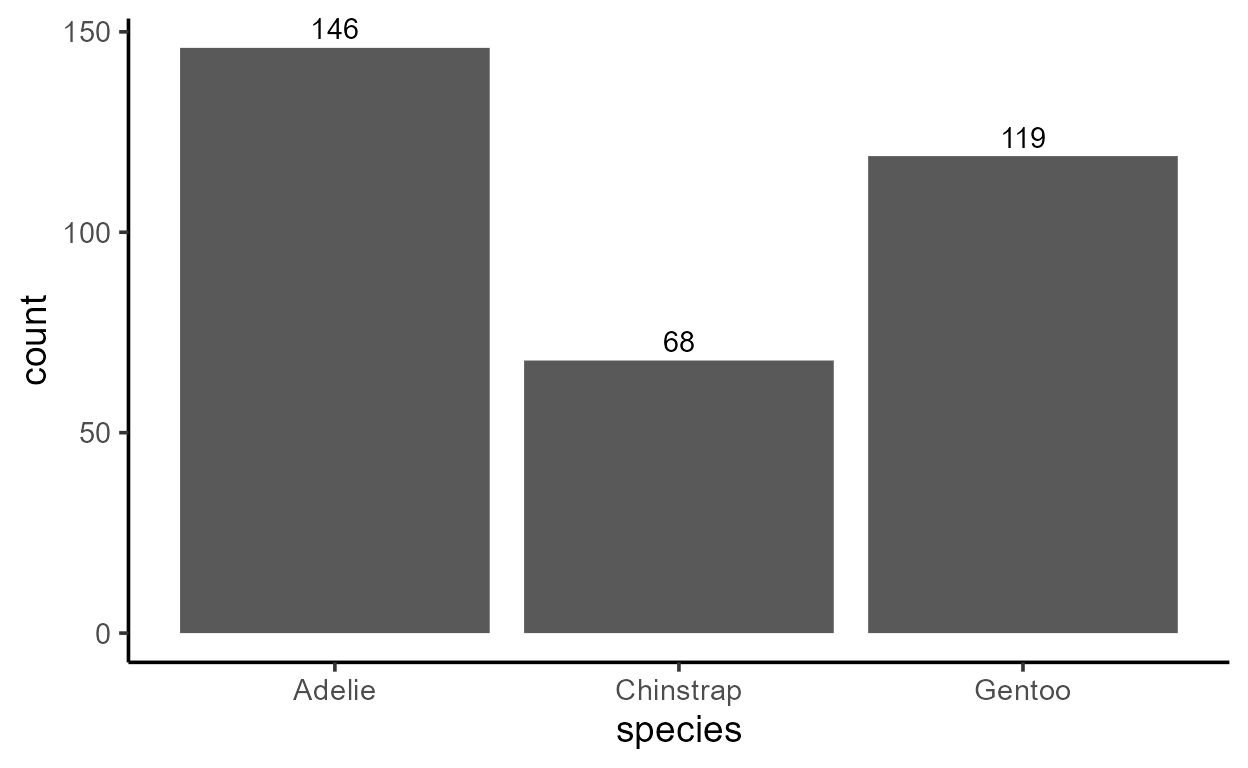But notice that you can do the same with two calls to `stat_count()`:

``````ggplot(penguins, aes(species)) +
stat_count(geom = "bar") +
stat_count(
aes(label = after_stat(count)),
geom = "text", vjust = -.4
)
``````I personally like the second style using `stat_count()` for two reasons:

1. When I read `stat_count()`, I know going in that the variable `count` is going to be available in the `after_stat()` for that layer, so I’m not surprised to see `label = after_stat(count)` in the `aes()` of the text layer.

2. It makes the code tell a coherent story - the theme of this plot is about visualizing counts, and what gets drawn to visualize counts is secondary to that overarching goal. This happens to also aligns pretty well with my actual data viz thought process.

Which do you prefer?

### Sneak peak of Part 2

I’ve tried my best to split up this topic of delayed aesthetic evaluation to keep it more manageable, so hopefully this wasn’t too overwhelming!

Part 2 will pick up where we left off by exploring when, where, and how the data actually undergoes the statistical transformation. We will do a lot more of “peaking inside” the internals similar to what we did with our custom function `inspect_after_stat()`, except we will use functions from my package `{ggtrace}` to interact with ggplots internals. It’s a package partly designed as a pedagogical tool for folks in early stages of the user-to-developer transition, and I’m very excited to showcase its potentials!

One last note: Part 2 will introduce the ggproto system which powers ggplot internals. It’s notoriously difficult to grasp, and has a big learning curve even if you’re an experienced user of ggplot. But fear not, because we already got a little taste of ggproto! Remember how we were just passing strings to the `geom` and `stat` arguments of layer functions? Well that was actually just a shorthand for specifying ggproto objects like `StatCount` and `GeomBar`:

``````ggplot(penguins, aes(species)) +
geom_bar(stat = StatCount) # same as `stat = "count"`

ggplot(penguins, aes(species)) +
stat_count(geom = GeomBar) # same as `geom = "bar"`
``````

We will talk more about these ggproto objects, especially `Stat` ggprotos like `StatCount`.

## Session Info

``````sessionInfo()
``````
``````  R version 4.1.1 (2021-08-10)
Platform: x86_64-w64-mingw32/x64 (64-bit)
Running under: Windows 10 x64 (build 19044)

Matrix products: default

locale:
 LC_COLLATE=English_United States.1252
 LC_CTYPE=English_United States.1252
 LC_MONETARY=English_United States.1252
 LC_NUMERIC=C
 LC_TIME=English_United States.1252

attached base packages:
 stats     graphics  grDevices utils     datasets  methods   base

other attached packages:
 dplyr_1.0.8   ggplot2_3.3.5

loaded via a namespace (and not attached):
 highr_0.8            pillar_1.6.4         bslib_0.3.1
 compiler_4.1.1       jquerylib_0.1.4      tools_4.1.1
 digest_0.6.28        downlit_0.4.0        jsonlite_1.7.2
 evaluate_0.14        memoise_2.0.0        lifecycle_1.0.1
 tibble_3.1.6         gtable_0.3.0         pkgconfig_2.0.3
 rlang_1.0.2          DBI_1.1.2            cli_3.1.1
 rstudioapi_0.13      distill_1.3          yaml_2.2.1
 xfun_0.29            fastmap_1.1.0        withr_2.4.2
 stringr_1.4.0        knitr_1.37           generics_0.1.0
 vctrs_0.3.8          sass_0.4.0           systemfonts_1.0.3
 tidyselect_1.1.1     grid_4.1.1           glue_1.6.1
 R6_2.5.1             textshaping_0.3.6    fansi_1.0.2
 rmarkdown_2.11       farver_2.1.0         purrr_0.3.4
 magrittr_2.0.1       palmerpenguins_0.1.0 scales_1.1.1
 htmltools_0.5.2      ellipsis_0.3.2       assertthat_0.2.1
 colorspace_2.0-2     labeling_0.4.2       ragg_1.2.0
 utf8_1.2.2           stringi_1.7.5        munsell_0.5.0
 cachem_1.0.1         crayon_1.4.2``````

1. I introduce `layer_data()` in a previous blog post on stat layers↩︎

2. Okay, not quite all the way down because you still have to make and draw graphical objects that actually make up the figure, but this is a substantial part of the internals and you get the point.↩︎

3. The label geom, by virtue of not having any groupings specified, is assigned a group value of -1 (see `ggplot2:::NO_GROUP`). This introduces another group category to the pre-existing groups 1 and 2 created by the bar layer, and so this has consequences for when the fill scale steps in to derive values of `fill` from the levels of `group`. ↩︎

4. Note also how `group` appears in different column positions. When `group` is explicitly supplied, it appears to the left of `PANEL`, and when `group` is derived it appears to the right of `PANEL`. Where and how does this happen? I’ll leave this question as an exercise (hint: look at `Layer\$compute_aesthetics` and `ggplot2:::add_group()`). ↩︎

5. You can replace `1` with any length-1 value - the point is to just not create any groupings.↩︎

6. Why not across facets? That’s a good question and has to do with how by-panel and by-group computations are done inside ggplot. We’ll look at this more in detail in Part 2!↩︎

7. As well as a “stack” position, but we won’t go into that in this blog post series.↩︎

8. Don’t you appreciate how you can just use `geom_histogram()` to draw a histogram without having to think about `stat = "bin"` every time?↩︎